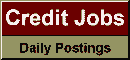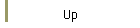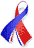DefaultRisk.com the web's biggest credit risk modeling resource.Conditional Expectation as Quantile Derivative

by Dirk Tasche of Technische Universität München

November 13, 2000

Abstract: For a linear combination of random variables, fix some confidence level and consider the quantile of the combination at this level. We are interested in the partial derivatives of the quantile with respect to the weights of the random variables in the combination. It turns out that under suitable conditions on the joint distribution of the random variables the derivatives exist and coincide with the conditional expectations of the variables given that their combination just equals the quantile. Moreover, using this result, we deduce formulas for the derivatives with respect to the weights of the variables for the so-called expected shortfall (first or higher moments) of the combination. Finally, we study in some more detail the coherence properties of the expected shortfall in case it is defined as a first conditional moment.

Keywords: quantile, value-at-risk, quantile derivative, conditional expectation, expected shortfall, conditional value-at-risk, coherent risk measure.